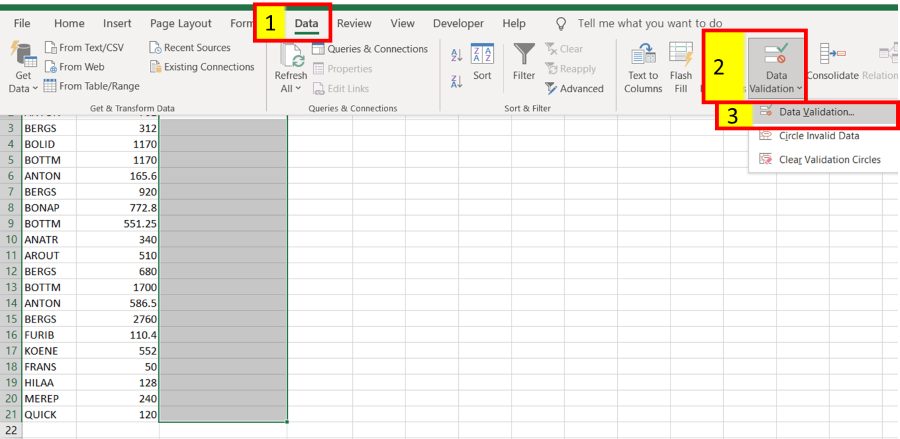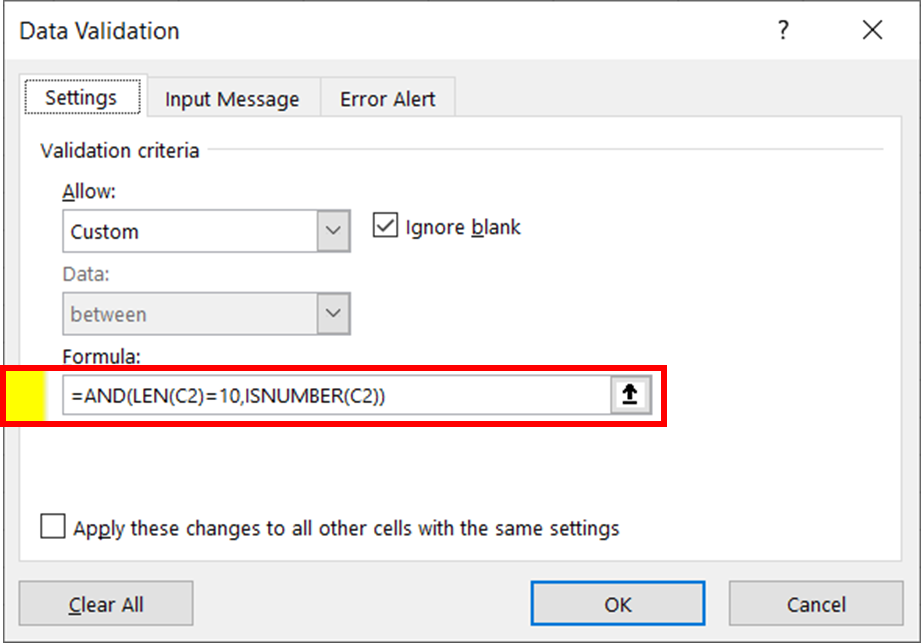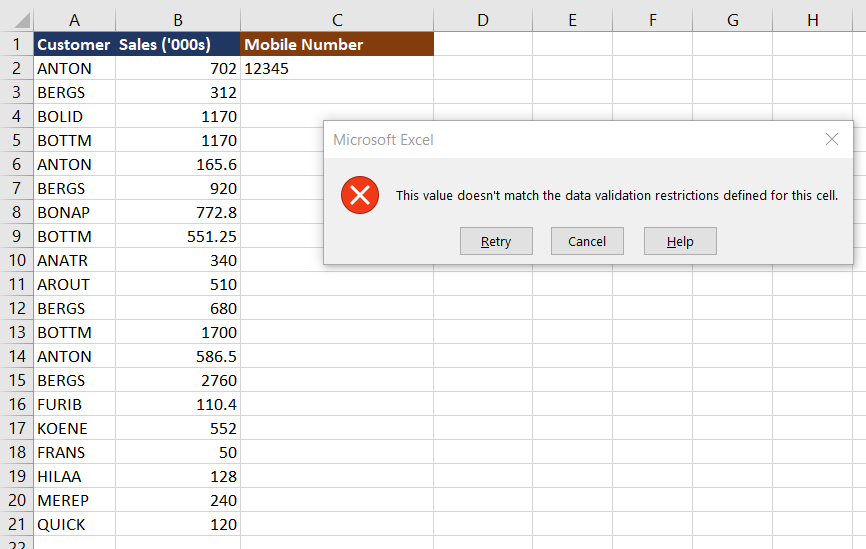# Use Custom Data Validation Function To Limit Number of Digits In Excel

• Last Updated : 29 Oct, 2021

There might arise a situation while working on Excel where you want the users to type in 10-digits(say)  numbers only in a cell. In these types of scenarios, we can make use of the Custom Data validation Function to limit the number of digits in Excel. In this article, we will discuss the same.

Sample Data: Customer Sales Table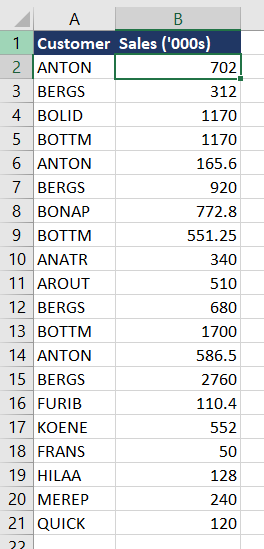Sales Table

Approach:

First, we check the given cell value is number and then the cell value is only 10 digit. Allow only both conditions are true

Excel Formula:

• ISNUMBER(): Return Boolean value True if the cell value is number.
• LEN(): Return the number of characters in a cell.
• AND(): Logical and function.

### Implementation of Custom Data Validation Function:

Follow the below steps to implement the custom data validation function:

Step 1: Type Header (“Mobile Number” as an example) in Cell C1.

Step 2: Select cells “C2” to “C21”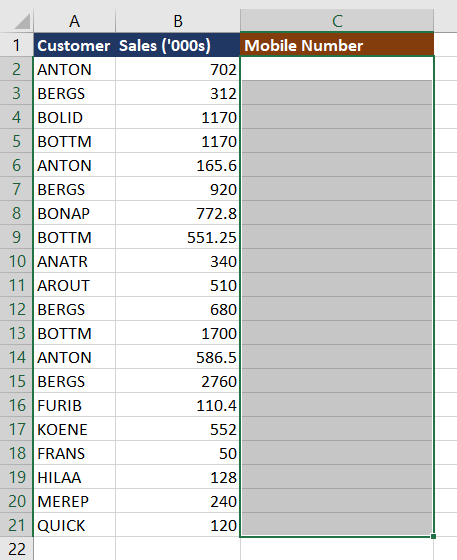Step 3: Click Data >> Data Validation >> Data Validation… to open the Data Validation dialog box as shown below. Here we will be implementing the function as :

`AND(LEN(C2)=10,ISNUMBER(C2))`